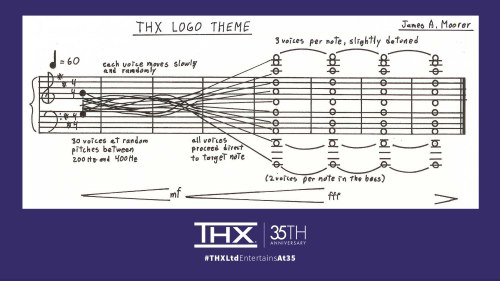# Mathematics of Deep NoteI just finished listening to the latest episode of Twenty Thousand Hertz, the story behind “Deep Note,” the THX logo sound.

There are a couple mathematical details of the sound that I’d like to explore here: random number generation, and especially Pythagorean tuning.

## Random number generation

First is that part of the construction of the sound depended on a random number generator. The voices start in a random configuration and slowly reach the target D major chord at the end.

Apparently the random number generator was not seeded in a reproducible way. This was only mentioned toward the end of the show, and a teaser implies that they’ll go more into this in the next episode.

## Pythagorean tuning

The other thing to mention is that the final chord is based on Pythagorean tuning, not the more familiar equal temperament.

The lowest note in the final chord is D1. (Here’s an explanation of musical pitch notation.) The other notes are D2, A2, D3, A3, D4, A4, D5, A5, D6, and F#6.

### Octaves

Octave frequencies are a ratio of 2:1, so if D1 is tuned to 36 Hz, then D2 is 72 Hz, D3 is 144 Hz, D4 is 288 Hz, D5 is 576 Hz, and D6 is 1152 Hz.

### Fifths

In Pythagorean tuning, fifths are in a ratio of 3:2. In equal temperament, a fifth is a ratio of 27/12 or 1.4983 , a little less than 3/2. So Pythagorean fifths are slightly bigger than equal temperament fifths. (I explain all this here.)

If D2 is 72 Hz, then A2 is 108 Hz. It follows that A3 would be 216 Hz, A4 would be 432 Hz (flatter than the famous A 440), and A5 would be 864 Hz.

### Major thirds

The F#6 on top is the most interesting note. Pythagorean tuning is based on fifths being a ratio of 3:2, so how do you get the major third interval for the highest note? By going up by fifths 4 times from D4, i.e. D4 -> A4 -> E5 -> B5 -> F#6.

The frequency of F#6 would be 81/16 of the frequency of D4, or 1458 Hz.

The F#6 on top has a frequency 81/64 that of the D# below it. A Pythagorean major third is a ratio of 81/64 = 1.2656, whereas an equal temperament major third is f 24/12 or 1.2599 . Pythagorean tuning makes more of a difference to thirds than it does to fifths.

A Pythagorean major third is sharper than a major third in equal temperament. Some describe Pythagorean major chords as brighter or sweeter than equal temperament chords. That the effect the composer was going for and why he chose Pythagorean tuning.

### Detuning

Then after specifying the exact pitches for each note, the composer actually changed the pitches of the highest voices a little to make the chord sound fuller. This makes the three voices on each of the highest notes sound like three voices, not just one voice. Also, the chord shimmers a little bit because the random effects from the beginning of Deep Note never completely stop, they are just diminished over time.

## Related posts

 The exponent is 7/12 because a half step is 1/12 of an octave, and a fifth is 7 half steps.

 The exponent is 4/12 because a major third is 4 half steps.

Musical score above via THX Ltd on Twitter.